﻿ Year 3 Addition & Subtraction (1)
 HOME ABOUT US SHOP PRIVACY CONTACT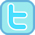Year 1 Year 2 Year 3 Year 4 Year 5 Year 6

# Year 3 Addition & Subtraction (1)

### Pupils should be taught to add and subtract numbers mentally including a three-digit number and ones, a three-digit number and tens, a three-digit number and hundreds.

 Our printable resources...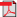Add 1-digit to 3-digit Mad Maths Minutes Sets A & B          Sets C & D          Sets A & B (black)          Sets C & D (black)Subtract 1-digit from 3-digit Mad Maths Minutes Sets A & B          Sets C & D          Sets A & B (black)          Sets C & D (black)Adding / Subtracting 1-digit to/from 3-digit Mad Maths Minutes Sets A & B          Sets C & D          Sets A & B (black)          Sets C & D (black)Adding multiple of 10 to 3-digit (multiples of 10) Mad Maths Minutes Sets A & B          Sets C & D          Sets A & B (black)          Sets C & D (black)Subtract Multiples of 10 from 3-digit (multiples of 10) MMM Sets A & B          Sets C & DSubtract Multiples of 10 from 3-digit (multiples of 10) MMM (black) Sets A & B          Sets C & DAdding / Subtracting multiples of 10 (3-digit mult. 10) MMM Sets A & B          Sets C & DAdding / Subtracting multiples of 10 (3-digit mult. 10) MMM (black) Sets A & B          Sets C & DAdding/Subtracting 10 to/from 3-digit Mad Maths Minutes Sets A & B          Sets C & D          Sets A & B (black)          Sets C & D (black)Adding multiple of 10 to 3-digit Mad Maths Minutes Sets A & B          Sets C & DAdding multiple of 10 to 3-digit Mad Maths Minutes (black) Sets A & B          Sets C & DSubtract Multiples of 10 from 3-digit Mad Maths Minutes Sets A & B          Sets C & DSubtract Multiples of 10 from 3-digit Mad Maths Minutes (black) Sets A & B          Sets C & DAdding / Subtracting multiples of 10 and 3-digit Mad Maths Minutes Sets A & B          Sets C & DAdding / Subtracting multiples of 10 and 3-digit Mad Maths Minutes (black) Sets A & B          Sets C & DAdding multiple of 100 to 3-digit Mad Maths Minutes Sets A & B          Sets C & DAdding multiple of 100 to 3-digit Mad Maths Minutes (black) Sets A & B          Sets C & DSubtract multiples of 100 from 3-digit Mad Maths Minutes Sets A & B          Sets C & DSubtract multiples of 100 from 3-digit Mad Maths Minutes (black) Sets A & B          Sets C & DAdding / Subtracting multiples of 100 and 3-digit Mad Maths Minutes Sets A & B          Sets C & DAdding / Subtracting multiples of 100 and 3-digit MMM (black) Sets A & B          Sets C & D
 Our online activities...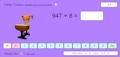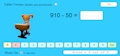Adding 1-digit to 3-digit Interactive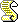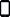Subtract 1-digit from 3-digit InteractiveAdding / Subtracting 1-digit and 3-digit InteractiveAdding multiples of 10 to 3-digit (multiples of 10) InteractiveSubtract mulitple of 10 from 3-digit (multiple of 10) InteractiveAdding / Subtracting 2-digit and 3-digit multiples of 10Adding multiples of 10 to 3-digit InteractiveSubtract multiples of 10 from 3-digit InteractiveAdding / Subtracting multiples of 10 and 3-digit numbers InteractiveAdding multiples of 100 to 3-digit InteractiveSubtracting multiples of 100 from 3-digit InteractiveAdding / Subtracting Multiples of 100 InteractiveLinks to sites that we like...